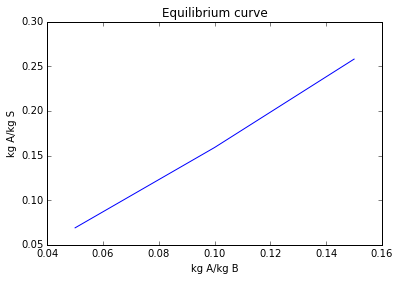# Chapter 13 - Liquid–Liquid Extraction¶

## Page 732 Example 13.1¶

In :
from sympy import symbols,solve
%matplotlib inline
from matplotlib.pyplot import plot,title,xlabel,ylabel,show

print"\n    (a) Countercurrent operation"
#(a) Countercurrent operation
S = 1.6*10**(-4)#               #Solvent flow rate in m**3/sec
mass_flowrate = (S*800)#       #mass flow rate is in kg/sec

#Considering the solution, 400cm3/s = 4 × 10−4 m**3/sec containing, say, a m**3/sec A and (5 × 10−4 − a) m3/sec B.
#Thus mass flow rate of A = 1200a kg/sec
#and mass flow rate of B = (4*10**(-4)-a)*1000 = (0.4-1000a)kg/sec
#a total of:                    (0.4+200a) kg/sec
C = symbols('C')#
C1 = solve(0.1*(0.4+200*C)-1200*C)
print"\n Concentration of the solution is %.2f*10**(-5) m**3/sec"%(C1*10**5)
print"\n mass flow rate of A = %.3f kg/sec"%(1200*C1)
print"\n mass flow rate of B =%.3f kg/sec"%(0.4+200*C1)
print"\n Ratio of A/B in the feed, Xf = %.3f kg/kg"%(0.041/0.366)

X = [0.05, 0.10, 0.15]
Y = [0.069 ,0.159, 0.258]
plot(X,Y)
title("Equilibrium curve")
xlabel("kg A/kg B")
ylabel("kg A/kg S")
show()
#From The curve:
slope = 0.366/0.128#
print"\n Slope of the equilibrium line is %.2f"%(slope)#

#Since pure solvent is added, Yn+1 = Y4 = 0 and a line of slope 2.86 is drawn in such that stepping off from Xf = 0.112 kg/kg to Y4 = 0 gives exactly three stages. When Y4 = 0, Xn = X3 = 0.057 kg/kg
print"\n The composition of final raffinate is 0.057kg A/kg B"

print"\n\n\n      (b)Multiple contact"
print"\n Stage 1"
print"\n In this case %.4f kg/sec"%(0.128/3)#
#and from the equilibrium curve, the extract contains 0.18 A/kg S and (0.18 × 0.0427) = 0.0077 kg/s A.
print"\n Thus raffinate from stage 1 contains %.4f kg/sec Aand %.3f kg/sec B"%((0.041-0.0077),0.366)
X1 = 0.0333/0.366#
print"\n X1 = %.3f kg/kg"%(0.0333/0.366)

print"\n Stage 2"
#the extract contains 0.14 kg A/kg S
print"\n The extract contains %.4f kg/sec A"%(0.14*0.0427)
#Thus: the raffinate from stage 2 contains (0.0333 − 0.0060) = 0.0273 kg/s A and 0.366 kg/s B
X2 = (0.0273/0.366)
print"\n X2 = %.3f kg/kg"%(X2)

print"\n Stage 3"
#the extract contains 0.114 kg A/kg S
print"\n The extract contains %.4f kg/sec A/kg S"%(0.114*0.0427)
print"\n Thus the raffinate contains %.4f kg/sec A and %.3f kg/sec B"%((0.0273-0.0049),0.366)
print"\n The composition of final raffinate = %3f kg A/kg B"%(0.0224/0.366)

    (a) Countercurrent operation

Concentration of the solution is 3.39*10**(-5) m**3/sec

mass flow rate of A = 0.041 kg/sec

mass flow rate of B =0.407 kg/sec

Ratio of A/B in the feed, Xf = 0.112 kg/kg

Slope of the equilibrium line is 2.86

The composition of final raffinate is 0.057kg A/kg B

(b)Multiple contact

Stage 1

In this case 0.0427 kg/sec

Thus raffinate from stage 1 contains 0.0333 kg/sec Aand 0.366 kg/sec B

X1 = 0.091 kg/kg

Stage 2

The extract contains 0.0060 kg/sec A

X2 = 0.075 kg/kg

Stage 3

The extract contains 0.0049 kg/sec A/kg S

Thus the raffinate contains 0.0224 kg/sec A and 0.366 kg/sec B

The composition of final raffinate = 0.061202 kg A/kg B## Page 736 Example 13.3¶

In :
from math import log

#From the equilibrium relationship
CB1 = (0.0247*0.685)#
print"\n CB1* = %.4f kmol/m**3"%(CB1)#
CB2 = (0.0247*0.690)#
print"\n CB2* = %.4f kmol/m**3"%(CB2)#

#Thus the driving force at the bottom:
deltaC1 = (0.0169-0.0040)#
print"\n deltaC1 =%.4f kmol/m**3"%(deltaC1)#
#Driving force at the top
deltaC2 = (0.0170-0.0115)#
print"\n deltaC2 = %.4f kmol/m**3"%(deltaC2)#
def log_mean_driving_force():
x = (deltaC1 - deltaC2)/log((deltaC1)/deltaC2)#
return x
print"\n log mean driving force is given by deltaClm = %.4f kmol/m**3"%(log_mean_driving_force())#
KBa = (4.125*10**(-8))/(log_mean_driving_force()*0.0063)#
print"\n KBa = %.1f*10**(-4) kmol/sec m**3(kmol/m**3)"%(KBa*10**4)#
HoB = (1.27*10**(-3))/KBa#
print"\n HoB = %.2f meters"%(HoB)#

 CB1* = 0.0169 kmol/m**3

CB2* = 0.0170 kmol/m**3

deltaC1 =0.0129 kmol/m**3

deltaC2 = 0.0055 kmol/m**3

log mean driving force is given by deltaClm = 0.0087 kmol/m**3

KBa = 7.5*10**(-4) kmol/sec m**3(kmol/m**3)

HoB = 1.68 meters


## Page 754 Example 13.4¶

In :
diameter = [2, 3 ,4 ,5, 6]#
number = [30, 120, 200, 80, 20]#

def Sum_d1cube():
sum = 0#
i = 0#
while (i <= 4):
sum = sum + number[i]*(diameter[i])**3#
i = i+1

x = sum
return x

def sum_d1square():
sum1 = 0
j=0
while(j<=4):
sum1 = sum1 + number[j]*(diameter[j])**2
j= j+1

y = sum1
return y

def ds():
z = Sum_d1cube()/sum_d1square()
return z
print"\n Mean droplet size = %.2f mm"%(ds())

 Mean droplet size = 4.00 mm


## Page 758 Example 13.5¶

In :
from math import pi

CSA = (pi/4)*(0.075)**2#             #cross sectional area is in m**2
V = (0.0044*3)#                      #volume of packing is in m**3
C = 0.01#                            #concentration is in kg/kg
print"\n mass of acid transferred to the ether %.4f kg/m**2.sec or %f kg/sec"%(0.05*(0.01-0),0.005*0.0044)#
print"\n Acid in the aqueous feed = %.2f kg/m**3.sec"%(0.25*0.04)#
print"\n Acid in the raffinate = %.3f kg/m**2.sec"%(0.01-0.005)#
print"\n Concentration of acid in the raffinate = %.2f kg/kg"%(0.005/0.25)#
print"\n At the top of the column"
CR2 = 0.040#               #Concentration is in kg/kg
CR22 = 0.040*0.3#          #Concentration is in kg/kg
deltaC2 = (0.012-0.010)#
print"\n deltaC2 = %.3f kg/kg"%(deltaC2)#
print"\n\n At the bottom of the column"
CR1 = 0.20#               #Concentration is in kg/kg
CR11 = 0.020*0.3          #Concentration is in kg/kg
deltaC1 = 0.006 -0#       #Concentration is in kg/kg
print"\n deltaC1 = %.3f kg/kg"%(deltaC1)#
deltaCRlm = (0.006-0.002)/log(0.006/0.002)#
print"\n Logarithmic driving force is : %.4f kg/kg"%(deltaCRlm)#
KRa = 0.000022/(0.01333*deltaCRlm)#
print"\n KRa = %.3f kg/m**3.sec(kg/kg)"%(KRa)#
print"\n Height of an overall transfer unit = %.2f m"%(0.25/KRa)#
print"\n The number of overall transfer units = %.2f "%(3/0.54)#

 mass of acid transferred to the ether 0.0005 kg/m**2.sec or 0.000022 kg/sec

Acid in the aqueous feed = 0.01 kg/m**3.sec

Acid in the raffinate = 0.005 kg/m**2.sec

Concentration of acid in the raffinate = 0.02 kg/kg

At the top of the column

deltaC2 = 0.002 kg/kg

At the bottom of the column

deltaC1 = 0.006 kg/kg

Logarithmic driving force is : 0.0036 kg/kg

KRa = 0.453 kg/m**3.sec(kg/kg)

Height of an overall transfer unit = 0.55 m

The number of overall transfer units = 5.56